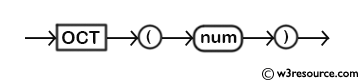# MySQL OCT() function

## OCT() function

MySQL OCT() returns octal value of a decimal number.

Syntax:

```OCT(num)
```

Argument

Name Description
num A decimal number whose octal representation is to be retrieved.

Syntax Diagram:MySQL Version: 5.6

Example: OCT() function

Code:

``````SELECT OCT('55');
```
```

Explanation

The above MySQL statement returns an octal representation of the decimal number 55.

Sample Output:

```mysql> SELECT OCT('55');
+-----------+
| OCT('55') |
+-----------+
| 67        |
+-----------+
1 row in set (0.03 sec)
```

All Mathematical FunctionsPrevious: MOD()
Next: PI()

﻿